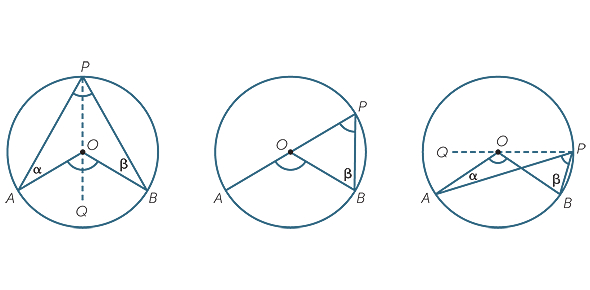# Circle Properties And Coordinate Geometry I

10 Questions | Total Attempts: 519SettingsRecall: tangent perpendicular to radius. tangents meet at a point equidistant from circumference. (isos. Triangle) alt seg thm angle at ctr = 2 angle at circumf. . . y-axis is x=0. So, lines parallel to y axis are x =? . . . x-axis is y=0. parallel lines have same gradient. product of gradient of perpendicular lines is -1.

• 1.
• A.

A

• B.

B

• C.

C

• D.

D

• 2.
• A.

A

• B.

B

• C.

C

• D.

D

• 3.
• A.

A

• B.

B

• C.

C

• D.

D

• 4.
• A.

A

• B.

B

• C.

C

• D.

D

• 5.
• A.

A

• B.

B

• C.

C

• D.

D

• 6.
• A.

A

• B.

B

• C.

C

• D.

D

• 7.
• A.

A

• B.

B

• C.

C

• D.

D

• 8.
• A.

A

• B.

B

• C.

C

• D.

D

• 9.
• A.

A

• B.

B

• C.

C

• D.

D

• 10.
• A.

A

• B.

B

• C.

C

• D.

D

Related TopicsBack to top